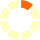# Ohio's Learning Standards: 4.MD.1

## Know relative sizes of the metric measurement units within one system of units. Metric units include kilometer, meter, centimeter, and millimeter; kilogram and gram; and liter and milliliter. Express a larger measurement unit in terms of a smaller unit. Record measurement conversions in a two-column table.For example, express the length of a 4-meter rope in centimeters. Because 1 meter is 100 times as long as a 1 centimeter, a two-column table of meters and centimeters includes the number pairs 1 and 100, 2 and 200, 3 and 300, ...* * Learning Farm includes imperial units in addition to metric units.<